# Rectangle - 8th grade (13y) - math problems

#### Number of problems found: 161

• A rectangle's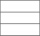A rectangle's length is 3 times more than twice its width. If the perimeter of the rectangle is 60 feet. What is the length of the rectangle
• RectangleThe width of the rectangle is 65% of its length. Perimeter of the rectangle is 132 cm. Determine the dimensions of the rectangle.
• Rectangle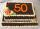Area of rectangle is 3002. Its length is 41 larger than the width. What are the dimensions of the rectangle?
• Rectangle - desc circle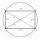Length of the sides of the rectangle are at a ratio 1: 3 . Radius of the circle circumscribed to rectangle is 10 cm. Calculate the rectangle's perimeter.
• Rectangle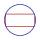The rectangle is 18 cm long and 10 cm wide. Determine the diameter of the circle circumscribed to the rectangle.
• RectangleIn a rectangle with sides, 6 and 3 mark the diagonal. What is the probability that a randomly selected point within the rectangle is closer to the diagonal than to any side of the rectangle?
• Circumscription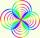Calculate radius of the Circumscribed circle in the rectangle with sides 20 and 19. It can be rectangle inscribed by circle?
• Rectangle 3-4-5The sides of the rectangle are in a ratio of 3:4. The length of the rectangle diagonal is 20 cm. Calculate the content of the rectangle.
• Rectangle areaThe length of a rectangle of x units is increased by 10% and its width of y units is increased by 15%. What is the ratio of the area of the old rectangle to the area if the new rectangle?
• Diagonal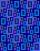Diagonal of the rectangle has length 46.4 cm. Angle between diagonal and londer sife of rectangle is 22°. Calculate area of the rectangle.
• Rectangle - parallelogram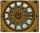It is given a rectangle that is circumscribed by a circle with a radius of 5 cm. The short side of the rectangle measures 6 cm. Calculate the perimeter of a parallelogram ABCD whose vertices are the midpoints of the sides of the rectangle.
• Inscribed rectangle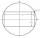The circle area is 216. Determine the area of inscribed rectangle with one side 5 long.
• RectangleThe length of the rectangle are in the ratio 5:12 and the circumference is 238 cm. Calculate the length of the diagonal and area of rectangle.
• Rectangle from stringString 12m. Make rectangle when one side is two times longer than its width.
• Desribed circle to rectangle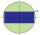Rectangle with sides 6 cm and 4 cm was circumscribed circle. What part of the content of the circle determined by the circumscribed circle occupies a rectangle? Express in perctentages(%).
• Enlarged rectangleThe rectangle with dimensions of 8 cm and 12 cm is enlarged in a ratio of 5:4. What are the circumference and the area of the enlarged rectangle?
• Rectangle - sides 4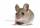Perimeter of the rectangle is 72 cm. Calculate the length of the sides that are in the ratio 3:5.
• ExpansionIf one side of the rectangle is larger 4-times and second 2-times, what percentage increases the area of rectangle?
• Square vs rectangleSquare and rectangle have the same area contents. The length of the rectangle is 9 greater and width 6 less than side of the square. Calculate the side of a square.
• RectangleIn rectangle ABCD with sides |AB|=19, |AD|=16 is from point A guided perpendicular to the diagonal BD, which intersects at point P. Determine the ratio ?.

Do you have an interesting mathematical word problem that you can't solve it? Submit a math problem, and we can try to solve it.

We will send a solution to your e-mail address. Solved examples are also published here. Please enter the e-mail correctly and check whether you don't have a full mailbox.

Please do not submit problems from current active competitions such as Mathematical Olympiad, correspondence seminars etc...